﻿ 近浅海单点浮筒式系泊系统的部分参数设计 A Parameter Design of a Single Point Buoy Type Mooring System near Shallow Sea

Advances in Applied Mathematics
Vol. 08  No. 05 ( 2019 ), Article ID: 30419 , 12 pages
10.12677/AAM.2019.85110

A Parameter Design of a Single Point Buoy Type Mooring System near Shallow Sea

Caiqiao Yi1, Haibing Sun2*, Yahan Yang1, Bin Li3, Xia Wang1

1School of Management, Sichuan Light Chemical University, Zigong Sichuan

2College of Mathematics and Statistics, Sichuan Light Chemical University, Zigong Sichuan

3College of Mechanical Engineering, Sichuan Light Chemical University, Zigong Sichuan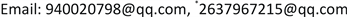Received: May 5th, 2019; accepted: May 20th, 2019; published: May 27th, 2019ABSTRACT

This paper focuses on the studies of mooring system parameters’ design. Firstly, the force analysis and torque analysis of the anchor chain, steel drum and heavy ball, steel pipe and buoy in the mooring system are carried out respectively. And the force balance equation and the moment balance equation are established. Then the buoy depth and the anchor chain are obtained, and the two expressions related to the horizontal angle of the left end tangent are finally obtained by MATLAB. The following results are obtained when the sea surface wind speed is 36. The inclination angle of the steel drum is 7.8566, the inclination angles of the four sections are 7.6962, 7.6557, 7.6156 and 7.5759, the draught-depth of the buoy is 0.7810 meters, and the area is 951.246. In addition, it is also discussed how to adjust the weight of the heavy ball such that the tilt angle of the steel drum is less than 5.

Keywords:Mooring System, Torque Balance, Catenary, Single Factor Control

1四川轻化工大学，管理学院，四川 自贡

2四川轻化工大学，数学与统计学院，四川 自贡

3四川轻化工大学，机械工程学院，四川 自贡Copyright © 2019 by authors and Hans Publishers Inc.1. 引言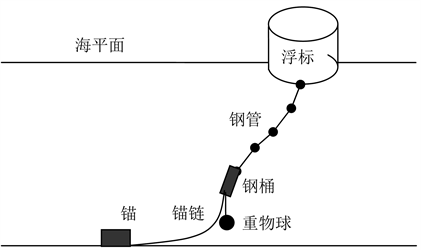Figure 1. Schematic diagram of the transmission node

2. 传输节点受力分析

2.1. 传输节点的整体水平受力分析如图2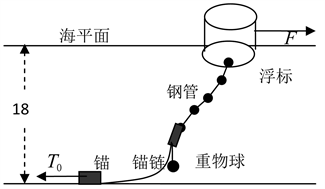Figure 2. Schematic diagram of the overall force of the transmission node

${T}_{0}=F$ (1-1)

$F=0.625×S{v}^{2}$ (单位：牛) (1-2)

$S=D×\left(H-h\right)$ (1-3)

$F=0.625{v}^{2}D\left(H-h\right)$ (1-4)

2.2. 单位悬链线静力矩分析及静力分析

$\left\{\begin{array}{l}F\left(T+\text{d}T,x\right)\ast L\left(T+\text{d}T,x\right)=F\left(T,x\right)\ast L\left(T,x\right)\\ F\left(T+\text{d}T,y\right)\ast L\left(T+\text{d}T,y\right)=F\left(T,y\right)\ast L\left(T,y\right)+G\left(T,{y}_{T}\right)\ast L\left(T,{y}_{T}\right)\end{array}$ (1-5)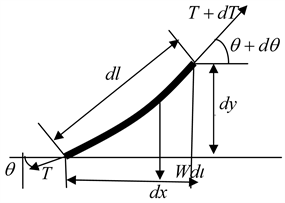Figure 3. Static diagram of the unit catenary

$\left\{\begin{array}{l}\text{d}x=\mathrm{cos}\theta \text{d}l\\ \text{d}y=\mathrm{sin}\theta \text{d}l\end{array}$ (1-6)

$\left(T+\text{d}T\right)\mathrm{cos}\left(\theta +\text{d}\theta \right)-T\mathrm{cos}\theta =0$ (1-7)

$\left(T+\text{d}T\right)\mathrm{sin}\left(\theta +\text{d}\theta \right)-T\mathrm{sin}\theta -W\text{d}l=0$ (1-8)

2.3. 完整悬链线静力分析与静力矩分析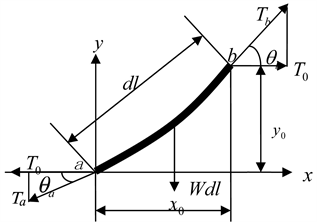Figure 4. Static diagram of the entire catenary

$\left\{\begin{array}{l}{T}_{0}={T}_{a}\mathrm{cos}{\theta }_{a}={T}_{b}\mathrm{cos}{\theta }_{b}\\ {T}_{bx}\ast {L}_{bx}={T}_{ax}\ast {L}_{ax}\\ {T}_{by}\ast {L}_{by}={T}_{ay}\ast {L}_{ay}+G\ast L\end{array}$ (1-9)

$x=\frac{{T}_{0}}{W}\left[{\mathrm{sinh}}^{-1}\left(\mathrm{tan}{\theta }_{b}\right)-{\mathrm{sinh}}^{-1}\left(\mathrm{tan}{\theta }_{a}\right)\right]$ (1-10)

$y=\frac{{T}_{0}}{W}\left(\sqrt{1+{\mathrm{tan}}^{2}{\theta }_{b}}-\sqrt{1+{\mathrm{tan}}^{2}{\theta }_{a}}\right)$ (1-11)

2.4. 悬链线方程求解

${t}_{a}={\mathrm{sinh}}^{-1}\left(\mathrm{tan}{\theta }_{a}\right)$${t}_{b}={\mathrm{sinh}}^{-1}\left(\mathrm{tan}{\theta }_{b}\right)$ 。将 ${t}_{a}$${t}_{b}$ 带入式(1-10)和式(1-11)中化简得到：

$x=\frac{{T}_{0}}{W}\left({t}_{b}-{t}_{a}\right)$ (1-12)

$y=\frac{{T}_{0}}{W}\left(\mathrm{cosh}{t}_{b}-\mathrm{cosh}{t}_{a}\right)$ (1-13)

${t}_{b}=\frac{xW}{T}+{t}_{a}$ (1-14)

$y=\frac{{T}_{0}}{W}\left[\mathrm{cosh}\left(\frac{Wx}{T}+{t}_{a}\right)-\mathrm{cosh}{t}_{a}\right]$ (1-15)

2.5. 悬链线水平长度随浮标吃水深度变化规律

$l={\int }_{0}^{{x}_{0}}\sqrt{1+{\left({y}^{\prime }\right)}^{2}}\text{d}x$ (1-16)

${y}^{\prime }=\mathrm{sinh}\left(\frac{Wx}{{T}_{0}}+{t}_{a}\right)=\mathrm{tan}{\theta }_{b}$ (1-17)

$l={\frac{{T}_{0}}{W}\mathrm{sinh}\left(\frac{Wx}{{T}_{0}}+{t}_{a}\right)|}_{0}^{{x}_{0}}$ (1-18)

$l=\frac{0.625{v}^{2}D\left(H-h\right)}{W}\left[\mathrm{sinh}\left(\frac{W{x}_{0}}{0.625{v}^{2}D\left(H-h\right)}+{t}_{a}\right)-\mathrm{sinh}\left({t}_{a}\right)\right]$ (1-19)

3. 钢桶受力分析

3.1. 钢桶静力矩分析

$\left\{\begin{array}{l}{F}_{2x}\ast {L}_{2x}={T}_{cx}\ast {L}_{cx}\\ {F}_{2y}\ast {L}_{2y}={T}_{cy}\ast {L}_{cy}+G\ast L\end{array}$ (2-1)

3.2. 钢桶受力分析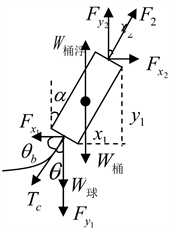Figure 5. Static analysis of steel drum

$\left\{\begin{array}{l}{F}_{2}\mathrm{sin}{\alpha }_{2}={T}_{c}\mathrm{sin}{\theta }_{c}\\ {F}_{2}\mathrm{cos}{\alpha }_{2}+{W}_{浮}={W}_{桶}+{T}_{c}\mathrm{cos}{\theta }_{c}+W\end{array}$ (2-2)

$\sum M=\sum \left(F×d\right)=0$ (2-3)

$-l\cdot {F}_{{y}_{2}}\cdot \mathrm{sin}{\alpha }_{1}+l\cdot {F}_{{x}_{2}}\cdot \mathrm{cos}{\alpha }_{1}+\frac{1}{2}{W}_{重\text{-}浮}\cdot \mathrm{sin}{\alpha }_{1}=0$ (2-4)

${l}_{桶}\mathrm{sin}{\alpha }_{1}\cdot {F}_{2}\mathrm{cos}{\alpha }_{2}+{l}_{桶}\mathrm{cos}{\alpha }_{1}\cdot {F}_{2}\mathrm{sin}{\alpha }_{2}+\frac{1}{2}W{l}_{桶}\mathrm{sin}{\alpha }_{1}=0$ (2-5)

$\mathrm{tan}{\alpha }_{1}=\frac{-{T}_{c}\cdot \mathrm{sin}{\theta }_{c}}{\frac{3}{2}\left({W}_{桶}-{W}_{浮}\right)+{W}_{球}-{T}_{c}\cdot \mathrm{cos}{\theta }_{c}}$ (2-6)

3.3. 钢管静力矩分析

$\left\{\begin{array}{l}{F}_{\left(n-1,x\right)}\ast {L}_{\left(n-1,x\right)}={T}_{\left(n+1,x\right)}\ast {L}_{\left(n+1,x\right)}\\ {F}_{\left(n-1,y\right)}\ast {L}_{\left(n-1,y\right)}+{F}_{浮n}\ast L={T}_{\left(n+1,y\right)}\ast {L}_{\left(n+1,y\right)}+{G}_{n}\ast L\end{array}$ (2-7)

3.4. 钢管受力分析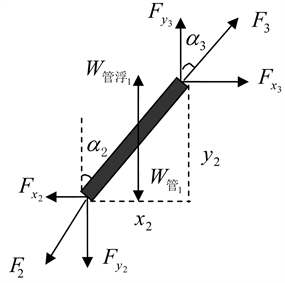Figure 6. Static analysis of steel pipe 1

$\left\{\begin{array}{l}{F}_{3}\mathrm{sin}{\alpha }_{3}={F}_{2}\mathrm{sin}{\alpha }_{2}\\ {F}_{3}\cdot \mathrm{cos}{\alpha }_{3}={F}_{2}\cdot \mathrm{cos}{\alpha }_{2}+{W}_{{管}_{1}}-{W}_{{浮}_{1}}\end{array}$ (2-8)

${F}_{3}^{2}={F}_{2}^{2}+{\left({W}_{{管}_{1}}-{W}_{{浮}_{1}}\right)}^{2}+2\left({W}_{{管}_{1}}-{W}_{{浮}_{1}}\right)\sqrt{{F}_{1}^{2}-{T}_{0}^{2}}$ (2-9)

${F}_{2}^{2}={T}_{c}^{2}+{\left({W}_{桶}-{W}_{桶浮}+{W}_{球}\right)}^{2}+2{T}_{c}\cdot \mathrm{cos}{\theta }_{c}\left({W}_{桶}-{W}_{桶浮}+{W}_{球}\right)$ (2-10)

4. 浮标受力分析

4.1. 浮标静力矩分析

$\left\{\begin{array}{l}{F}_{\left(4,x\right)}\ast {L}_{\left(4,x\right)}={F}_{风}\ast L\\ {F}_{\left(4,y\right)}\ast {L}_{\left(4,y\right)}+G\ast L={F}_{浮}\ast L\end{array}$ (3-1)

4.2. 浮标受力分析

$F=0.625×D\left(H-h\right){v}^{2}$ (3-2)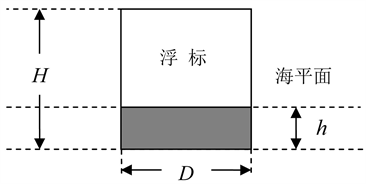Figure 7. Schematic diagram of the buoy on the projection plane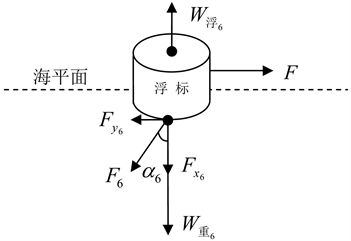Figure 8. Static analysis of the buoy

$\left\{\begin{array}{l}F={F}_{6}\mathrm{sin}{\alpha }_{6}=0.625D\left(H-h\right){v}^{2}\\ {F}_{6}\mathrm{cos}{\alpha }_{6}+{W}_{{重}_{6}}={W}_{{浮}_{6}}=\frac{1}{4}\rho gh\text{π}{D}^{2}\end{array}$ (3-3)

5. 系泊系统整体分析

5.1. 系泊系统受力分析

$\left\{\begin{array}{l}F={F}_{6}\mathrm{sin}{\alpha }_{6}\\ {F}_{6}\mathrm{sin}{\alpha }_{6}={F}_{5}\mathrm{sin}{\alpha }_{5}\\ {F}_{5}\mathrm{sin}{\alpha }_{5}={F}_{4}\mathrm{sin}{\alpha }_{4}\\ {F}_{4}\mathrm{sin}{\alpha }_{4}={F}_{3}\mathrm{sin}{\alpha }_{3}\\ {F}_{3}\mathrm{sin}{\alpha }_{3}={F}_{2}\mathrm{sin}{\alpha }_{2}\\ {F}_{2}\mathrm{sin}{\alpha }_{2}={T}_{c}\mathrm{sin}{\theta }_{c}\\ {T}_{c}\mathrm{sin}{\theta }_{c}={T}_{0}\end{array}$ (4-1)

5.2. 系泊系统竖直长度分析

$Y={y}_{0}+{l}_{桶}\mathrm{cos}{\alpha }_{1}+{l}_{管}\mathrm{cos}{\alpha }_{2}+{l}_{管}\mathrm{cos}{\alpha }_{3}+{l}_{管}\mathrm{cos}{\alpha }_{4}+{l}_{管}\mathrm{cos}{\alpha }_{5}+h$ (4-2)

5.3. 浮标游动区域的确定

$\left\{\begin{array}{l}L={x}_{0}+{l}_{桶}\mathrm{sin}{\alpha }_{1}+{l}_{管}\mathrm{sin}{\alpha }_{2}+{l}_{管}\mathrm{sin}{\alpha }_{3}+{l}_{管}\mathrm{sin}{\alpha }_{4}+{l}_{管}\mathrm{sin}{\alpha }_{5}\\ S=\text{π}{L}^{2}\end{array}$ (4-3)

6. 模型求解

  

step1：将锚链左下端切线与海床的夹角 ${\theta }_{a}$ 和锚链水平长度 ${x}_{0}$ 带入(1-17)，计算出锚链对钢桶张力的方向与水平线的夹角 ${\theta }_{b}$

step2：由图4可知 ${\theta }_{b}$${\theta }_{c}$ 互余，求得 ${\theta }_{c}$ ；根据(1-6)可以求得 ${T}_{b}$ 。因为 ${T}_{b}$${T}_{c}$ 为一对相互作用力，可计算出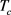的值。

step3：将 ${T}_{c}$${\theta }_{c}$ 带入(2-5)，(2-12)，分别计算出钢桶倾斜角度 ${\alpha }_{1}$${F}_{2}$

step4：将 ${T}_{c}$${\theta }_{c}$${F}_{2}$ 带入式(3-3)，计算出钢管1的倾斜角度 ${\alpha }_{2}$ ，钢管2的倾斜角度 ${\alpha }_{3}$ ，钢管3的倾斜角度 ${\alpha }_{4}$ ，钢管4的倾斜角度 ${\alpha }_{5}$ ，锚链张力沿水平方向分力 ${T}_{0}$

step5：将 ${\theta }_{a}$${T}_{0}$ 带入(1-11)，求得悬链线方程；利用Matlab做出锚链的形状图。

step6：将锚链水向长度 ${x}_{0}$ ，钢管1~钢管4的倾斜角 ${\alpha }_{2}$ ~ ${\alpha }_{4}$ 带入(4-3)，求解出系泊系统水平长度L，浮标游动的区域面积S。即系泊系统浮标的游动区域是以锚为原点，半径为L的圆，浮标游动区域面积为S。Table 1. Tilt angle of steel drum and each section of steel pipe when sea surface wind speed is 36 (unit: ˚C), buoy draft depth (unit: m)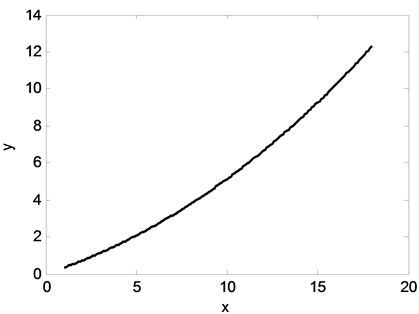Figure 9. Anchor chain shape when the sea surface wind speed is 36

${y}_{3}=\frac{1974.8}{7}\left[\mathrm{cosh}\left(\frac{7x}{1974.8}+0.3171\right)-1.0507\right]$ (5-1)

$\left\{\begin{array}{l}{W}_{重}={F}_{2}\mathrm{cos}{\alpha }_{2}+{W}_{浮}-{W}_{桶}-{T}_{c}\mathrm{cos}{\theta }_{c}\\ {F}_{2}\mathrm{cos}{\alpha }_{2}=\sqrt{{F}^{2}-{F}^{2}{\mathrm{sin}}^{2}{\alpha }_{2}}=\sqrt{{F}_{2}^{2}-{T}_{0}^{3}}\\ \frac{1}{\mathrm{tan}{\theta }_{c}}=\mathrm{tan}{\theta }_{b}=\mathrm{sinh}\left(\frac{W{x}_{0}}{T}+{t}_{a}\right)\end{array}$ (5-2)

${W}_{重}=\sqrt{{F}_{2}^{2}-{T}_{0}^{2}}+{W}_{浮}-{W}_{桶}-\frac{{T}_{0}}{\mathrm{tan}{\theta }_{c}}$ (5-3)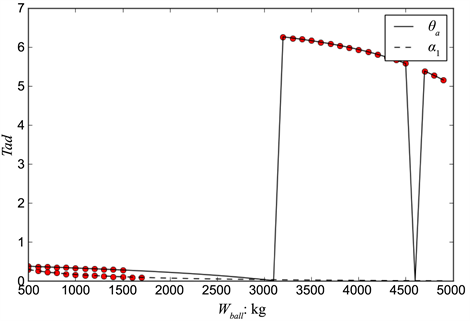Figure 10. ${\alpha }_{1}$ and ${\theta }_{a}$ the variation of the weight of the weight ball ${F}_{w}$

7. 结果分析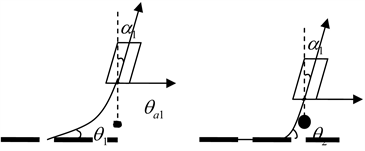Figure 11. Schematic diagram of the catenary of different weight balls

A Parameter Design of a Single Point Buoy Type Mooring System near Shallow Sea[J]. 应用数学进展, 2019, 08(05): 965-976. https://doi.org/10.12677/AAM.2019.85110

1. 1. 李玉龙, 于定勇, 李翠琳, 常梅. 防风单点系泊系统系泊力试验研究[J]. 海岸工程, 2008, 27(2): 17-25.

2. 2. 王领, 刘生法. 深水大型浮台系泊系统水动力计算分析[J]. 广东造船, 2018(5): 16-18.

3. 3. 孙传耀, 汤鸣晓. 基于MATLAB的系泊系统的设计及其动力分析[J]. 电子技术与软件工程, 2017(6): 62-63.

4. 4. 李继锁, 朱克强, 孙培军, 王晨. 船舶系泊系统动力分析[J]. 舰船科学技术, 2014, 36(10): 64-67.

5. 5. 刘文玺, 周其斗, 张纬康, 纪刚. 浮体与系泊系统的耦合动力分析[J]. 船舶力学, 2014, 18(8): 940-957.

6. 6. 马巍巍, 范模. 极浅水单点系泊系统的设计研究[J]. 船舶工程, 2013, 35(2): 92-95.

7. 7. 金伟锋, 吴思佳, 章春豪, 徐嘉琦, 徐俊康, 金书玉. 近浅海环境监测系泊系统的设计研究[J]. 湖南理工学院学报(自然科学版), 2017, 30(1): 7-10.

8. 8. 罗晓健, 张爱霞, 李绂. 单点系泊系统设计思路及原则[J]. 中国海洋平台, 2013, 28(2): 19-23+56.

9. 9. 袁梦, 范菊, 缪国平, 朱仁传. 系泊系统动力分析[J]. 水动力学研究与进展A辑, 2010, 25(3): 285-291.

10. 10. 夏运强, 唐筱宁, 蒋凯辉, 王绿卿. 防风单点系泊系统系泊力理论计算公式研究[J]. 水运工程, 2010(4): 58-62.

11. 11. 李淑一, 王树青. 基于多体分析的浅水FPSO和水下软钢臂系泊系统运动特性研究[J]. 中国海洋大学学报, 2011, 41(9): 95-102.

12. 12. 王瀚, 解德. 悬链线构件的有限元方法[J]. 中国舰船研究, 2015, 10(6): 34-38.

13. 13. 唐友刚, 张若瑜, 庄茁. 深海系泊系统模态分析[J]. 工程力学, 2010, 27(1): 233-239.

14. 14. 刘建成, 李润培, 顾永宁, 张剑波. 一种简易单点系泊系统的可行性研究[J]. 上海交通大学学报, 2000, 34(1): 132-136.

15. 15. 谭丰林, 许海城, 谌子民, 唐西林. 基于模拟退火的近浅海系泊系统仿真[J]. 现代电子技术, 2018, 41(2): 1-5.

16. NOTES

*通讯作者。